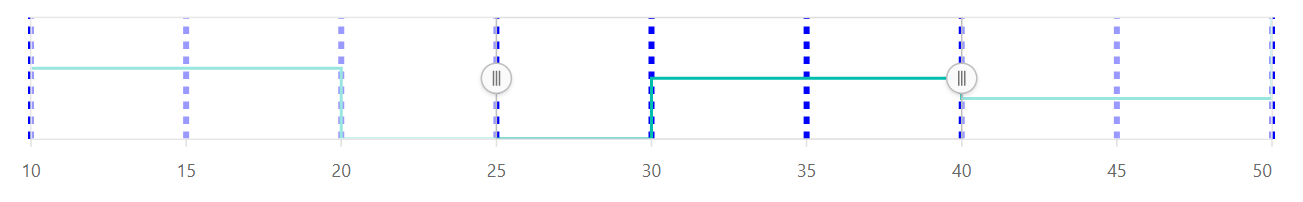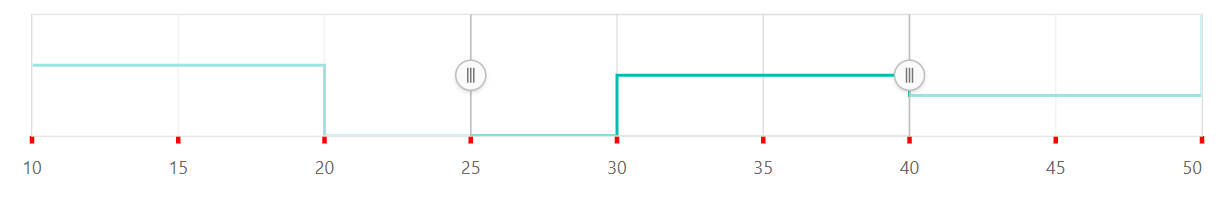# Grid and Tick Lines

17 Feb 20226 minutes to read

## Grid line customization

The gridlines indicate axis divisions by drawing the chart plot. Gridlines include helpful cues to the user, particularly for large or complicated charts. The `width`, `color`, and `dashArray` of the major gridlines can be customized by using the `majorGridLines` setting.

``````@(Html.EJS().RangeNavigator("container")
.ValueType(Syncfusion.EJ2.Charts.RangeValueType.DateTime)
.LabelFormat("{value}K")
.MajorGridLines(ViewBag.lineWidth)
.Series(sr =>
{
}).Render()
)``````
``````public IActionResult Index()
{
List < data>dataSource = new List<data>
{
new data {  y = 12, y1 = 28 },
new data {  y = 34, y1 = 44 },
new data {  y = 45, y1 = 48 },
new data {  y = 56, y1 = 50 },
new data {  y = 67, y1 = 66 },
new data {  y = 78, y1 = 78 },
new data {  y = 89, y1 = 84 },
};
ViewBag.dataSource = dataSource;
return View();
}
public class data
{
public double y;
public double y1;
}``````## Tick line customization

Ticklines are the small lines which is drawn on the axis line representing the axis labels. Ticklines will be drawn outside the axis by default. The `width`, `color`, and `dashArray` of the major ticklines can be customized by using the `majorTickLines` setting.

``````@(Html.EJS().RangeNavigator("container")
.ValueType(Syncfusion.EJ2.Charts.RangeValueType.DateTime)
.LabelFormat("{value}K")
.MajorTickLines(ViewBag.lineWidth)
.Series(sr =>
{
}).Render()
)``````
``````public IActionResult Index()
{
List < data>dataSource = new List<data>
{
new data {  y = 12, y1 = 28 },
new data {  y = 34, y1 = 44 },
new data {  y = 45, y1 = 48 },
new data {  y = 56, y1 = 50 },
new data {  y = 67, y1 = 66 },
new data {  y = 78, y1 = 78 },
new data {  y = 89, y1 = 84 },
};
ViewBag.dataSource = dataSource;
return View();
}
public class data
{
public double y;
public double y1;
}``````